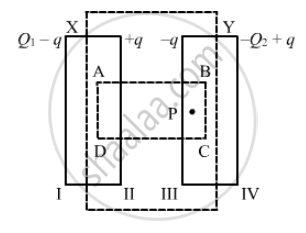Department of Pre-University Education, Karnataka course PUC Karnataka Science Class 12
Share

# P Suppose a Charge +Q1 is Given to the Positive Plate and a Charge −Q2 to the Negative Plate of a Capacitor. What is the "Charge on the Capacitor"? - Physics

ConceptCombination of Capacitors

#### Question

Suppose a charge +Q1 is given to the positive plate and a charge −Q2 to the negative plate of a capacitor. What is the "charge on the capacitor"?

#### SolutionGiven :

Charge on the positive plane = +Q_1

Charge on the negative plate = -Q_2

To calculate: Charge on the capacitor

Let ABCD be the Gaussian surface such that faces AD and BC lie inside plates X and Y, respectively.
Let q be the charge appearing on surface II. Then, the distribution of the charges on faces I, III and IV will be in accordance with the figure.

Let the area of the plates be A and the permittivity of the free space be ∈_0.

Now, to determine q​ in terms of Q1 and Q2, we need to apply Gauss's law to calculate the electric field due to all four faces of the capacitor at point P. Also, we know that the electric field inside a capacitor is zero.

Electric field due to face I at point P, E1 = (Q_1 - q)/(2∈_0A)

Electric field due to face II at point P, E2= (+q)/(2∈_0A)

Electric field due to face III at point P, E3 = (-q)/{2∈_0A)

Electric field due to face IV at point P, E4 = -((-Q_2+q)/(2∈_0A))      (Negative sign is used as point P lies on the LHS of face IV.)

Since point P lies inside the conductor,

E1 + E2 + E3 + E4 = 0

therefore Q_1-q+q-q-(-Q_2+q) = 0

⇒ q = (Q_1+Q_2)/2

Thus , the change on the capacitor is (Q_1+Q_2)/2, which is the charge on faces  II and III.

Is there an error in this question or solution?

#### APPEARS IN

HC Verma Solution for Concepts of Physics - Vol. 2 (2018 to Current)
Chapter 9: Capacitors
Short Answers | Q: 1 | Page no. 163

#### Video TutorialsVIEW ALL 

Solution P Suppose a Charge +Q1 is Given to the Positive Plate and a Charge −Q2 to the Negative Plate of a Capacitor. What is the "Charge on the Capacitor"? Concept: Combination of Capacitors.
S